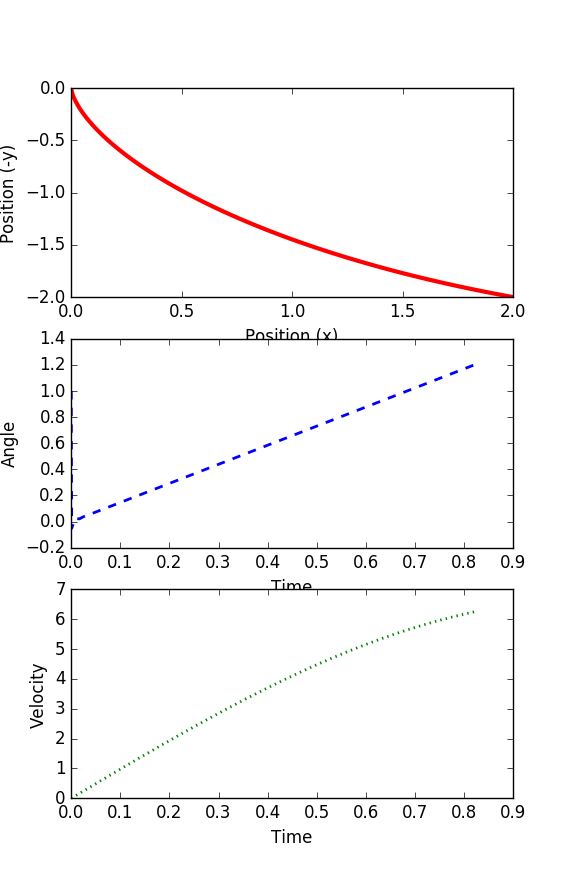## Brachistochrone Optimal Control

A classic optimal control problem is to compute the brachistochrone curve of fastest descent. A point mass must slide without friction and with constant gravitational force to an fixed end point in the shortest time. The following animation (source: Wikipedia) shows different trial solutions (blue) and the optimal solution (red) for a particular starting and end point.This is a classic problem that has been solved with calculus of variations but this particular task is to use a numerical method to solve this same problem. The solution curve is independent of both gravitational force and the mass of the object. The solution is different if there is an initial velocity or if there is friction.

#### Problem Statement

The adjustable parameter u is the slope and can be adjusted over the minimized time horizon tf. The variable x is the horizontal position and y is the vertical position in the down direction while v is the velocity. The parameter g is the gravitational constant (assume 9.81 m/s2).

$$\min t_f$$

$$\mathrm{subject\;to}$$

$$\quad \frac{dx(t)}{dt} = v \; \sin(u)$$

$$\quad \frac{dy(t)}{dt} = v \; \cos(u)$$

$$\quad \frac{dv(t)}{dt} = g \; \cos(u)$$

$$\quad x(0)=0, \quad y(0)=0, \quad v(0)=0$$

$$\quad x(t_f)=2, \quad y(t_f)=2, \quad v(t_f)=Free$$

#### SolutionDownload Brachistochrone Solution in PythonAn interesting extension to this problem is to compare the solutions to when the value of x is varied from 1.0 to 10.0. The final value of y is fixed at 2.0 below the starting point.Download Brachistochrone Parametric Solution in PythonThanks to Trent Okeson for providing a solution to this problem.